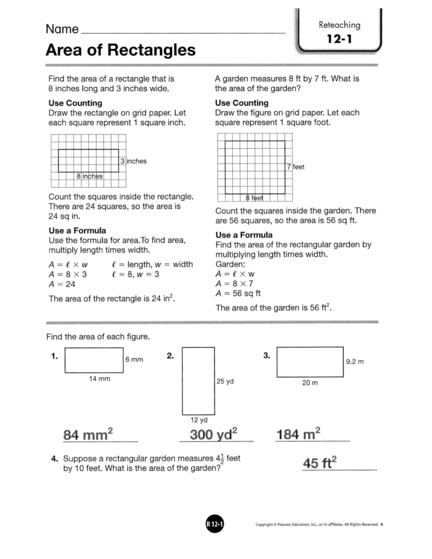# Finding Area of Rectangles and ParallelogramContributed by:This pdf helps us to find the area of rectangles and parallelograms by using counting and formula. Since base × height gives the area of the rectangle, we can use the same measurements on the parallelogram to compute its area: base × height.
1.
2.
3.
4.
5.
6.
7.
8.
9.
10.
11.
12.
13.
14.
15.
16.
17.
18.
19.
20.
21.